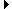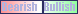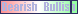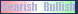Members AreaSign Up | Log In
 Free Level 2 QuotesStock AnalysisCorrelation Trading PlatformCorrelationsMembership Benefits

EMA
 Lookup a different indicator.

 Indicator Usage Description Example ema ema[x,n] OPERATOR (INDICATOR or POSITIVE NUMBER) , where x is a positive integer >= 2 and n is optional and represents the number of days ago the result is computed (n=0 is the default value and represents the current end of day, n=1 is the previous end of day, etc.) Returns the x-day exponential moving average closing price from n days ago. (close < ema) and (close > ema)

Introduction:

The calculation of the EMA (exponential moving average) places more weight on recent prices. The 'ema' indicator is used in indicator formula construction to narrow the stock pre-screener results to include only those stocks that have an exponential moving average stock price restricted to the parameters set for the ema indicator.

Example:

The chart of Intel (INTC) below uses the above example indicator to highlight days where the closing price was below the 40-day exponential moving average, yet was above the 40-day ema the prior day.

 Chart of Intel (INTC) highlighting days where the closing price was below the 40-day exponential moving average, yet was above the 40-day ema the prior day.

INDICATORS IN OUR DATABASE THAT INCLUDE THE EMA INDICATOR...

Click here to view all indicators that include the indicator 'ema' (sorted by AISCORE)

 ((macd[12,26,9] / ema) > 0.05)AISCORE: 470Indicator ID: 051226200903((macd[12,26,9] / ema) < -0.05)AISCORE: 3337Indicator ID: 051226200947((macd[12,26,9] / ema) < -0.02)AISCORE: 6503Indicator ID: 051226201037((macd[12,26,9] / ema) < -0.03)AISCORE: 7570Indicator ID: 051226201159((macd[12,26,9] / ema) < -0.04)AISCORE: 3488Indicator ID: 051226201300 More ->